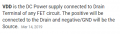# What is exactly explanation for power consumption which is the formula is P=IV OR P=I(VDD)*VDD

#### aryna

Joined Dec 10, 2020
19
hi everyone,
Can you guys explain more about the power consumption, im a little bit confuse, after reading too much to made my understanding better

#### Papabravo

Joined Feb 24, 2006
19,570
Your title question is meaningless because they are both the same. Power, in any element of a circuit, can be computed in any one of three ways, and they all are equivalent and give the same result.
1. If you know the current through a device and it's resistance then $$P\;=\;I^2 \cdot R$$
2. If you know the voltage across a device and the resistance then $$P\;=\;\frac {V^2}{R}$$
3. If you know the voltage across a device and the current through the device then $$P\;=\;V\cdot I$$
Each of these relationships follows from Ohm's law and one of the others. In cases 1 & 2 you can see that for a resistor the power cannot be negative. To get the same result in case three you must choose the positive value for the current which flow from the higher potential to the lower potential. Loads dissipate power, sources supply that power.

#### Ramussons

Joined May 3, 2013
1,321

#### jpanhalt

Joined Jan 18, 2008
11,088
Technically VDD is:There is also VCC, which relates to the Collector of a BJT circuit.

I practice either is used to represent the DC supply voltage for a circuit. A circuit with a voltage regulator might have VBAT -->voltage regulator -->VDD or VCC for the rest of the circuit. However, if you look at various schematics across the internet or even on this site, the rigorous definitions are not strictly observed. ECAD programs, like Eagle, will remind you when the rigorous definition is violated.

#### Deleted member 115935

Joined Dec 31, 1969
0
Power
is joules per second.

How does that help... ?

In a resistance circuit the power is defined by the Volts times amps across the load. W = VI

If you know the resistance,

Then from ohms law you know volts = Amps times resistance. V=IR

Which with some maths , allows you to substitute one into the other.

Note , the volts mentioned is the volts dropped across the load,

So if you have two resistances in series, each resistance has its own voltage drop and as such its own power dissipation.

W=VI
W = V*V/R
W= I*I*R

#### ericgibbs

Joined Jan 29, 2010
16,761
hi aryna,

As stated, your two versions give the same result.

W = Volts * Amps

E

#### djsfantasi

Joined Apr 11, 2010
8,652
I’m missing something here... The TS gave two equations:
P=IV
P=IV^2​
They aren’t equal.

#### ericgibbs

Joined Jan 29, 2010
16,761
hi dj,
This what the TS was asking
whiCh is the formula is P=IV OR P=I(VDD)*VDD

He had been told in his other thread they are same when using his LTS circuit

E

•djsfantasi

#### Ramussons

Joined May 3, 2013
1,321
Technically VDD is:
View attachment 227161
There is also VCC, which relates to the Collector of a BJT circuit.

I practice either is used to represent the DC supply voltage for a circuit. A circuit with a voltage regulator might have VBAT -->voltage regulator -->VDD or VCC for the rest of the circuit. However, if you look at various schematics across the internet or even on this site, the rigorous definitions are not strictly observed. ECAD programs, like Eagle, will remind you when the rigorous definition is violated.
I know what VDD generally means. I was stumped by the question that brings in terms that have simply no reference. Without a schematic, it can mean anything.I still don't know what I*(VDD)*VDD means#### crutschow

Joined Mar 14, 2008
31,112

#### jpanhalt

Joined Jan 18, 2008
11,088
I know what VDD generally means. I was stumped by the question that brings in terms that have simply no reference. Without a schematic, it can mean anything.I still don't know what I*(VDD)*VDD meansIt is pretty clear that the TS is using VDD to be synonymous with V.

#### BobaMosfet

Joined Jul 1, 2009
2,053

#### ericgibbs

Joined Jan 29, 2010
16,761
Where did you see that?

Hi C,
It's from his other Thread, got himself mixed up trying to use LTSpice models etc ..
E

#### neonstrobe

Joined May 15, 2009
181
It is pretty clear that the TS is using VDD to be synonymous with V.
I(VDD) is just current, written as a function of the power supply voltage. So it is just I, measured in the power line VDD.

#### neonstrobe

Joined May 15, 2009
181
I know what VDD generally means. I was stumped by the question that brings in terms that have simply no reference. Without a schematic, it can mean anything.I still don't know what I*(VDD)*VDD meansI(VDD) is just current, measured in the supply line. It should not be written I*(VDD).

•djsfantasi

#### djsfantasi

Joined Apr 11, 2010
8,652
I(VDD) is just current, measured in the supply line. It should not be written I*(VDD).
Now I get it... Never saw that notation convention used before, so I was a little confused. So the two equations ARE the same given your explanation of your notation.

#### Ramussons

Joined May 3, 2013
1,321